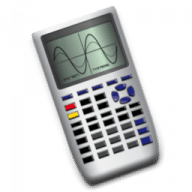Graphmatica
2.4

0.0

Graphmatica# Graphmatica2.4

01 December 2014

Equation plotter.

## Overview

Graphmatica is a powerful, easy-to-use equation plotter with numerical and calculus features. Graph Cartesian functions, relations, and inequalities, plus polar, parametric, and ordinary differential equations. Numerically solve and graphically display tangent lines and integrals. Find critical points, solutions to equations, and intersections between Cartesian functions. In summary, a great tool for students and teachers of anything from high-school algebra through college calculus.

## What's new in Graphmatica

Version 2.4:
##### New and improved:
• Curves are now highlighted as you mouse over them or select them in the equation list.
• Added Pick Line Style to the context menu for equations, allowing you to choose from solid, dashed, dotted, and dash-dot line styles.
• Fixed-increment Cartesian, polar, and parametric graphs (i.e. with the step rate specified as a third parameter in the domain) now display as a series of points; point tables are aligned to display the same values.
• The parser now accepts functions run together with a single-variable parameter, e.g. y=logx.
• Added support for specifying powers of functions before the parameter e.g. y=sin^2x or y=cos²x
• Any equation/inequality that is explicitly specified as x=f(y) is now graphed as a function of y, even if it can be solved for y instead.
Bug fixes
• Fixed bug which could cause graphs to be drawn slightly past the end of their specified domain.
• Find All Graphs now adjusts the range based only on the requested domain of the equations (rather than the maximum possible values that would be visible on the screen with an unrestricted domain).
• Selection highlighting now uses a dark transparent overlay (instead of a hard to see white overlay) for bright colors other than pure white.
• Fixed a number of issues with graphing inequalities like x < 1/y, where it is important to evaluate asymptotes as a function of y instead of x.
• Fixed a problem which caused ODEs (and implicit Cartesian functions) to fail to register (and later disappear) when evaluation failed due to a non-fatal error (overflow, out of domain, etc.). They now just stop drawing at that point.
• Eliminated extra vertical asymptote drawn as part of y=acot x.
• Fixed cubert(x) function to be defined for x < 0.

#### Join over 500,000 subscribers.

Subscribe for our newsletter with best Mac offers from MacUpdate.

How would you rate Graphmatica app?

Dylan-Mc
01 December 2014
Version: 2.4